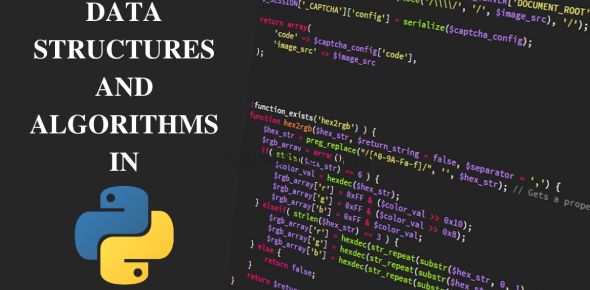# Data Structure And Algorithm Quiz: Test!

10 Questions | Attempts: 1685SettingsAre you familiar with data structure and algorithms? This quiz can help you to test your knowledge regarding both. A data structure is a named location that can be employed to keep and arrange the information. The algorithm is a series of steps to solve a specific problem. Learning data structures and algorithms allow us to write efficient and optimized computer programs. An algorithm is a step-by-step procedure that requires a set of instructions. The quiz below lets you test your memory about these two computer terminologies.

• 1.
Suppose you have a directed graph representing all the flights that an airline flies. What algorithm might be used to find the best sequence of connections from one city to another?
• A.

A shortest-path algorithm.

• B.

A minimum spanning tree algorithm.

• C.

• D.

Depth first search.

• 2.
Suppose cursor points to a node in a linked list (using the node definition with member functions called data and link). What Boolean expression will be true when the cursor points to the tail node of the list?
• A.

• B.

(cursor->data( ) == 0.0)

• C.

(cursor->data( ) == NULL)

• D.

(cursor == NULL)

• 3.
Which of the following represents the sequence of nodes visited in a post-order traversal of the binary tree T shown below?
• A.

U X Z Q W Y V P

• B.

X Z U W Y Q V P

• C.

U X W Q Z Y V P

• D.

U Q X W P V Z Y

• 4.
What does a run-time analysis usually count?
• A.

The number of arithmetic and other operations required for the program to run

• B.

The number of seconds plus the number of megabytes

• C.

The number of seconds required for the program to run

• D.

The number of megabytes required for the program to run

• 5.
Figure 2 is the array representation of a binary tree shown in Figure 1. What should be put into space "a"?
• A.

3

• B.

5

• C.

4

• D.

2

• 6.
Consider the following pseudo code: declare a stack of characters while ( there are more characters in the word to read ) { read a character push the character on the stack } while ( the stack is not empty ) { write the stack's top character to the screen pop a character off the stack } What is written to the screen for the input "cartets"?
• A.

Ccaarrtteettss

• B.

Cartets

• C.

Serc

• D.

Stetrac

• 7.
Given a graph G in the box. What is the order of nodes visited using DFS, starting from node a
• A.

A b c d e f k g h i j l

• B.

A c b g l k h i j f e d

• C.

A f e d c k g b h i j l

• D.

A c d e f k b g h i j l

• 8.
Which of the following stack operations could result in stack underflow?
• A.

Top

• B.

Pop

• C.

Is_empty

• D.

Push

• 9.
Which of the following is an appropriate description concerning the list and/or array structures?
• A.

Using a subscript for each element in an array, quick access to any element can be achieved. The array structure allows any data to be inserted or deleted simply by modifying pointers.

• B.

The number of operations is fixed in inserting or deleting an element in an array; it does not depend on the position of the element in the array

• C.

The list structure is similar to the array structure in that all data elements of the same type are sequentially lined up. In the list structure, the logical arrangement is the same as the physical arrangement.

• D.

The list structure allows any data to be inserted or deleted simply by modifying pointers. But, after the data was deleted, the cells that contained the data remain as garbage in memory

• 10.
There are two important operations on a stack: PUSH and POP. PUSH adds the new data to the top of the stack leaving previous data below, and POP removes and returns the current top data of the stack. When the operations shown below are sequentially executed, which of the following is the correct combination of the values x and y? Here, the size of the stack is big enough to hold the entire data. “PUSH(a)” inserts the data a into the stack, and “POP(b)” removes the data b from the stack. [Operations] PUSH (5); PUSH (3); PUSH (6); PUSH (1); x = POP (  ); PUSH (7); y = POP (  ); What are the value of X and Y?
• A.

• B.

• C.Back to top